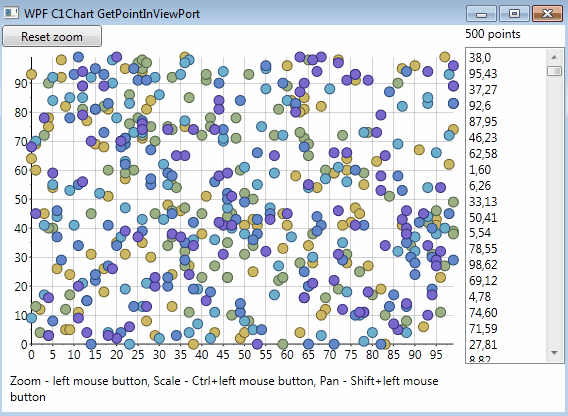﻿ C1Chart for WPF : Get Points in View on End User Interaction# C1Chart for WPF : Get Points in View on End User Interaction

ComponentOne Chart for WPF allows us to perform End User Interaction wherein we can zoom/ scale/ translate chart for a better view. As the title suggests, this blog explains the approach to get a list of all the points in the viewable area on Zooming, Scaling or Translating the ComponentOne Chart for WPF. The image below demonstrates the behavior discussed in this blog :WPF Chart Get Points In View To implement this, the GetPointsInView() method is used which returns the list of points as Data-Coordinates when any interaction (Zoom/Scale/Translate) is performed by the end-user. Here is the code that can be used :

``````
List<Point> GetPointsInView(C1Chart chart)
{
var list = new List<Point>();
int nser = chart.Data.Children.Count;
var xmin = GetAxisMin(chart.View.AxisX);
var xmax = GetAxisMax(chart.View.AxisX);
var ymin = GetAxisMin(chart.View.AxisY);
var ymax = GetAxisMax(chart.View.AxisY);

for (int i = 0; i < nser; i++)
{
var ds = chart.Data.Children[ i ] as XYDataSeries;
if (ds != null)
{
var values = ((IDataSeriesInfo)ds).GetValues();
if (values != null)
{
var len = values.GetLength(1);
for (int ipts = 0; ipts < len; ipts++)
{
var x = values[1, ipts];
var y = values[0, ipts];
if (!double.IsNaN(x) && !double.IsNaN(y))
{
if (xmin <= x && x <= xmax && ymin <= y && y <= ymax)
}
}
}
}
}
return list;
}

``````